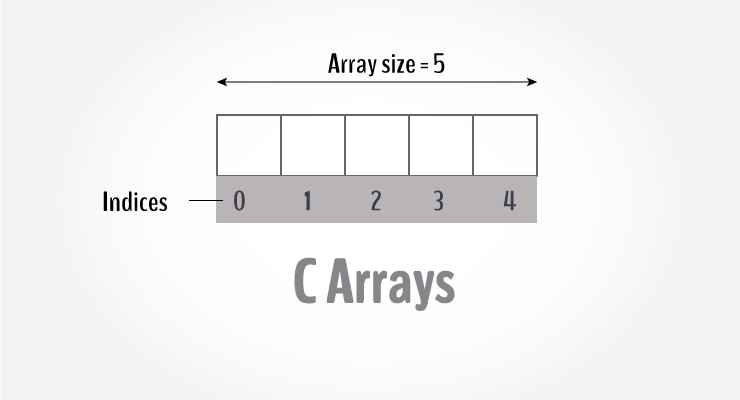# Why Array Indexing Starts with 0 in C?

Why array indexing starts with 0 in C, C++, Java and most of other programming languages is one of the common confusion among programmers.Image Source

## Why Array Indexing Starts with 0 in C?

Lets try to understand this by taking one example in C. We can declare an array of size 5 in following way.

int a;

Here a itself is a pointer which contains the memory location of first element of the array.

Now for accessing the first element we will write a which is internally decoded by compiler as *(a + 0).

In the same way the second element can be accessed by a or *(a + 1). As a contains the address of first element so for accessing second element we have to add 1 to it. Thats why here we have written *(a +1).

In array the index describes the offset from the first element, i.e. the distance from the first element.

Now lets assume that array indexing starts with 1 instead of 0. In this case for accessing the first element we have to write a which is internally decoded as *(a + 1 – 1).

Observe here that we have to perform one extra operation i.e. subtraction by 1. This extra operation will greatly decrease the performance when the program is big.

Thats why to avoid this extra operation and improve the performance, array indexing starts with 0 in C, C++, Java, etc.

Comment below if you have any queries regarding above article. Do share it if you found this information valuable.

### 5 thoughts on “Why Array Indexing Starts with 0 in C?”

1.Saloni Mulathia

ya …. i understand deeply and nice explanation.

2.Yes that’s quite natural. More over any number system always start from 0. It is our fault that we never taught like that way in nursery. Most of us never understand/asks our self why 10 comes after 9,100 comes after 99 etc .

Great article. 🙂 Hope this will help lots of students.

3.Great, but if this is the reason, so why in some other language, they start indexing from 1, e.g. MATLAB. Does not they concern about extra subtract operation like this?
Thank and best regards.

4.So, why other language, .e.g Matlab, they use 1-index notation?
Thanks and best regards,

5.Very nice article!

The nice thing is clear explanation with simple words.

Keep up the good work brother!